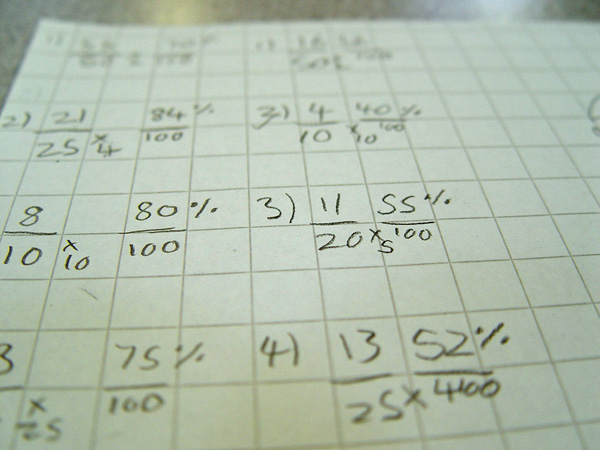# Year 11 MathsAll Year 11 pupils are continuing to make progress throughout the maths syllabus as we move ever closer to GCSE exam time. Our teaching staff are confident that some pupils are capable of achieving good results in the GCSE Foundation exams. We are also pleased that other pupils will be able to take part in the GCSE Maths Entry Level.

Each week we are looking at different areas of the curriculum. The students have just completed some work learning about how to convert fractions into a percentage.

Different methods were explained to help each student find a way of working that they feel comfortable with. Year 11 were shown how the basic theory behind this conversion involves multiplying the denominator by an amount that will give us 100. We then need to multiply the numerator by the same amount to convert our fraction to a percentage.

Each Year 11 student progressed through a maths worksheet to help solve some of the problems. An example might be to convert two fifths to a percentage.

We found that there are 20 fives in 100. Therefore we need to also multiply two by 20 to give a final percentage of 40.

All pupils were given adult support to help them with their work. Our calculations were set out on squared paper so that we could make sure that the tens, units and 100’s weren’t being mixed up. We encouraged all students to show their workings out.

Year 11 will continue to look at all areas covered in the GCSE maths curriculum before we decide which exam each student will sit.